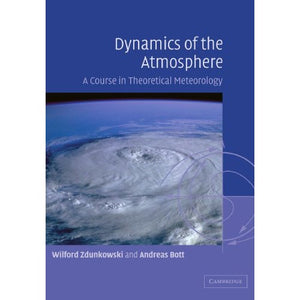># Dynamics of the Atmosphere: A Course in Theoretical Meteorology

• £18.99
• Save £60

Wilford Zdunkowski
Cambridge University Press, 4/10/2003
EAN 9780521006668, ISBN10: 052100666X

Paperback, 738 pages, 24.4 x 17 x 4.2 cm
Language: English

Dynamics of the Atmosphere consists of two parts: the first presenting the mathematical tools needed for a thorough understanding of the topics covered in the second part of the book. The second part begins with the derivation of the equation describing the atmospheric motion on the rotating earth. Subjects tackled in subsequent chapters include kinematics of the atmosphere (including vorticity and circulation theorems), wave motion in the atmosphere, inertial and dynamic stability, and turbulent systems in the atmosphere. Finally, newer methods of weather prediction, such as the spectral technique and the stochastic dynamic method, are introduced in order to demonstrate their potential for extending the forecasting range. Complete with numerous exercise sets and solutions, this textbook has been written for advanced undergraduate and graduate students of meteorology and other related sciences. It may also be used as a reference source by professional meteorologists and researchers in atmospheric science.

Preface
M1. Algebra of vectors
M2. Vector functions
M3. Differential relations
M4. Coordinate transformations
M5. The method of covariant differentiation
M6. Integral operations
M7. Introduction to the concepts of nonlinear dynamics
1. The laws of atmospheric motion
2. Scale analysis
3. The material and the local description of flow
4. Atmospheric flow fields
5. The Navier-Stokes stress tensor
6. The Helmholtz theorem
7. Kinematics of two-dimensional flow
8. Natural coordinates
9. Boundary surfaces and boundary conditions
10. Circulation and vorticity theorems
11. Turbulent systems
12. An excursion into the spectral turbulence theory
13. The atmospheric boundary-layer
14. Wave motion in the atmosphere
15. The barotropic model
16. Rossby waves
17. Inertial and dynamic stability
18. The equation of motion in general coordinate systems
19. The geographical coordinate system
20. The stereographic coordinate system
21. Orography-following coordinate systems
22. The stereographic system with a generalised vertical coordinate
23. A quasi-geostrophic baroclinic model
24. A two-level prognostic model
baroclinic instability
25. An excursion into numerical procedures
26. Modeling of atmospheric flow by spectral techniques
27. Predictability